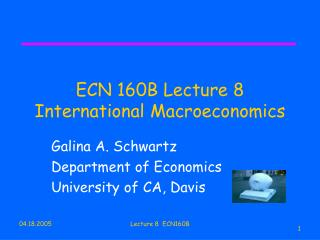Download PresentationECN 160B Lecture 8 International Macroeconomics

# ECN 160B Lecture 8 International Macroeconomics - PowerPoint PPT PresentationDownload Presentation## ECN 160B Lecture 8 International Macroeconomics

- - - - - - - - - - - - - - - - - - - - - - - - - - - E N D - - - - - - - - - - - - - - - - - - - - - - - - - - -
##### Presentation Transcript

1. ECN 160B Lecture 8International Macroeconomics Galina A. Schwartz Department of Economics University of CA, Davis Lecture 8 ECN160B

2. Brief on Money (next lecture) • Currencies = Monies • Money as a store of value, KO, p. 334 this is one of the functions of money • Demand for Currency ~ Demand for Money • Demand for Currency – two components • Demand by domestic residents • Demand by foreign residents • Supply of Currency (next lecture & next chapter) Lecture 8 ECN160B

3. Value of Currency • Currency is an asset • How to determine asset returns? (Or in which asset should one invest?) All else equal, one should invest into the asset with the highest real rate of return • As we have mentioned, asset value depends on ● Liquidity & ● Risk Lecture 8 ECN160B

4. ForEX Market Characteristics • Rate of Return (+ we will relate this to arguments about Interest Rate Parity(IRP)) • Expected ??? • Real ? What does this mean? • All else equal, in equilibrium, real returns on all assets should be equal, but • Risk (variability of returns) • Liquidity (cost (ease) & speed of selling the asset) • What affects liquidity? Ex. Market volume Lecture 8 ECN160B

5. Interest Rates & Asset Returns ●Rate of Return & ● Liquidity & ● Risk Rate of Return depends on two factors: • I. Interest rate that the currency offers (examples: R€ and R\$) • Interest rate is ? • II. Expected currency exchange rate against other currencies (ex. Ee\$/€) Lecture 8 ECN160B

6. Interest Rate Parity (IRP) • IRP: return on \$ deposits in terms of €: R€ +[(Ee\$/ € - E\$/€ )/E\$/€ ] = R\$ has to be equal to R\$ (otherwise profits can be made via arbitrage) KO, 13-2 • Therate of depreciationof thedollar against the euro is (E1\$/ € - E\$/€ )/E\$/€ E1\$/ € dollar/euro rate in one year Ee\$/ € expected dollar/euro rate in one year Lecture 8 ECN160B

7. FOREX Market Equilibrium • Equilibrium Determination: • Assumptions R€ , R\$ and Ee\$/€ are given (i.e., fixed) • Main conclusion: FOREX markets adjust to make deposits of all currencies offer the same expected rate of return • I.e., in equilibrium, IRP holds (KO, 13-2) Lecture 8 ECN160B

8. Exchange rate, E\$/€ Return on dollar deposits 2 E2\$/€ 1 E1\$/€ 3 E3\$/€ Expected return on euro deposits R\$ Rates of return (in dollar terms) ForEx Market Equilibrium Figure 13-4: Determination of the Equilibrium Dollar/Euro Exchange Rate Lecture 8 ECN160B

9. Exchange rate, E\$/€ Dollar return 1 1' E1\$/€ E2\$/€ 2 Expected euro return R1\$ R2\$ Rates of return (in dollar terms) Interest Rates, Expectations & Equilibrium Effect of a Rise in R\$ on E\$/€ Figure 13-5: Effect of a Rise in the Dollar Interest Rate Lecture 8 ECN160B

10. Exchange rate, E\$/€ Dollar return Rise in euro interest rate 2 E2\$/€ E1\$/€ 1 Expected euro return R\$ Rates of return (in dollar terms) Interest Rates, Expectations & Equilibrium: Effect of a rise in R€ on E\$/€ Figure 13-6: Effect of a Rise in the Euro Interest Rate Lecture 8 ECN160B

11. Non-covered versus Covered Interest Rate Parity • Non-covered interest rate parity – the parity involves the expected future spot exchange rate • Covered interest rate parity – involves parity condition based on forward exchange rate (not on the expected future spot exchange rate) Lecture 8 ECN160B

12. Next Lecture • Money • Long run • Short run • Equilibrium • Connection with interest rates • Exchange Rates Overshooting (time permitting) • covered • uncovered • Your preparation: KO Ch. 14 Lecture 8 ECN160B

13. Summary of Today • Rate of return • Rate of depreciation / appreciation • Interest parity condition aka Interest Rate Parity (IRP) • FOREX market equilibrium; How equilibrium changes with R or E • Equilibrium in the foreign exchange market requires interest parity. • For given interest rates and a given expectation of the future exchange rate, the interest parity condition tells us the current equilibrium exchange rate. • A rise in dollar (euro) interest rates causes the dollar to appreciate (depreciate) against the euro. • Today’s exchange rate is altered by changes in its expected future level. • Have a Nice Day Lecture 8 ECN160B